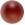Back

Body fat formula

From www.bmi-calculator.net

The body fat percentage function on home weight scales are of suspect accuracy. They work on resistance to electricity flowing through the body from one of the metal pads to the other. If like me you don't wear shoes much, the skin on your foot will be thicker. The following method will probably give a better figure.

The best time to use this formula is in the morning. Your body weight and waist measurements are the most accurate just after you wake up from 7-8 hours of sleep

The formula below will not calculate your exact body fat percentage. The most accurate way is underwater weighing, but this formula should give you a consistent measurement you can use as a guideline so you can determine if you're losing body fat and/or muscle.

Body Fat Formula For Women

Factor 1

(Total body weight x 0.732) + 8.987

Factor 2

Wrist measurement (at fullest point) / 3.140

Factor 3

Waist measurement (at navel) x 0.157

Factor 4

Hip measurement (at fullest point) x 0.249

Factor 5

Forearm measurement (at fullest point) x 0.434

Lean Body Mass

Factor 1 + Factor 2 - Factor 3 - Factor 4 + Factor 5

Body Fat Weight

Total bodyweight - Lean Body Mass

Body Fat %

(Body Fat Weight x 100) / total bodyweight

Body Fat Formula For Men

Factor 1

(Total body weight x 1.082) + 94.42

Factor 2

Waist measurement x 4.15

Lean Body Mass

Factor 1 - Factor 2

Body Fat Weight

Total bodyweight - Lean Body Mass

Body Fat %

(Body Fat Weight x 100) / total bodyweight

(C) Peter Scott 2015

Last edit 15 April 2017